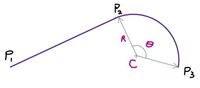Calculate Centre, Radius and Sweep angle of arc given start point, tangent line of start point and end point

ScottCal

New member
I am writing a computer program to plot a multi-segment path following the user's mouse movements. The user will choose one of 3 segment types: Line, Arc or Curve (Bezier). Each new segment will join tangentially to the preceding segment. My question is: how do I calculate the the centre, radius and sweep angle of of an arc starting tangentially to the preceding segment and passing through the user defined end point?Points P1, P2 and P3 are known. I need to calculate C, R and Θ ?

Attachments

• 15.5 KB Views: 2

Otis

Senior Member
Hello. I've assumed that you're using an xy-coordinate system. Have you learned enough algebra to find the equation of the straight line which passes through points P1 and P2 (using their known coordinates)?

I'm not sure where you got stuck, in this project …ScottCal

New member
Hi Otis,

Yes I am using an XY coordinate system and yes I can find the equation of the line passing through P1 and P2. I could also find the equation of the perpendicular line at the tangent point P2. So I know that the centre point C must lie on that line and therefore if I could calculate the radius or the angle between the line P1P2 and the tangent to the circle at P3 I could calculate the other values. This is where I am stuck! Alternatively if I could calculate the equation of the tangent line at P3, I could calculate the intersection point of the 2 tangents from which I could calculate the angle between the 2 lines and hence the angle Θ. However, I cannot see how to do this with an unknown centre point. Hope you can point me in the right direction.

Thanks.

Attachments

• 19.2 KB Views: 0

Dr.Peterson

Elite Member
You know that $$\displaystyle C$$ is on the line $$\displaystyle P_2C$$, whose equation you can find; you also know that $$\displaystyle C$$ lies on the perpendicular bisector of $$\displaystyle P_2P_3$$, right? Intersect those, and you have $$\displaystyle C$$. (And if it happens that $$\displaystyle P_3$$ lies on $$\displaystyle P_2C$$, $$\displaystyle C$$ is the midpoint of $$\displaystyle P_2P_3$$.)

ScottCal

New member
Thanks Dr Peterson, I can solve it now!! I did manage to come up with my own solution which is to equate P2C and P3C using pythagorus. I can then solve the resulting quadratic equation by substituting for Cx or Cy using the equation of P2C. However, I think your method will be more efficient because it doesn't involve solving a quadratic equation! Cheers.

Last edited:

Dr.Peterson

Elite Member
Actually, now that I think of it, if you carry out your method the right way, it reduces to my method, giving the equation of the bisector (and not actually requiring a quadratic equation). Or at least that's what I recall from handling similar problems in the past.